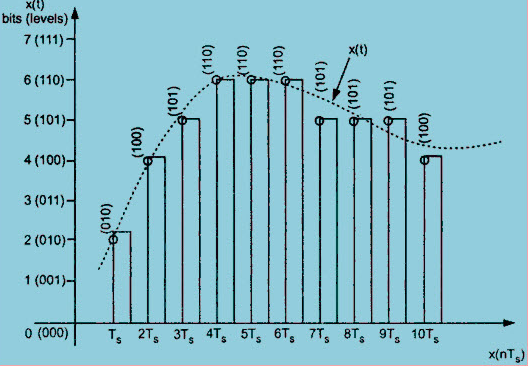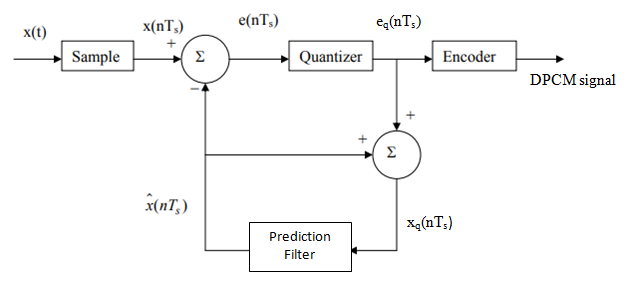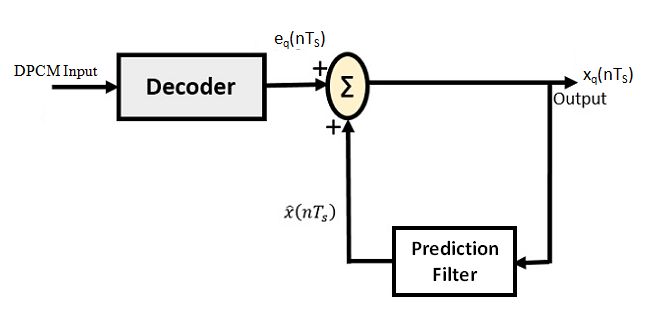# Explain Differential Pulse Code Modulation

## Differential Pulse Code Modulation

It may be observed that  the samples of a signal are highly correlated with each other. This is due to the fact that any signal does not change fast.  Which means , its value from present sample to next sample does not vary by a large amount.

The adjacent samples of the signal carry the same information with a little difference.

When these samples are encoded by a standard PCM system, the resulting encoded signal contains some redundant information.

### Redundant Information in PCM

Fig.1 shows a continuous time signal x(t) by dotted line. This signal is sampled by flat top sampling at intervals T, 2T, 3T …..  nTs .Fig.1 : Illustration of redundant information in PCM

The sampling frequency is selected to be higher than nyquist rate.

The samples are encoded by using 3 bit (7 levels) PCM.

The sample is quantized to the nearest digital level as shown by small circles in fig.1 .

The encoded binary value of each sample is written on the top of the samples.

We can observe from fig.1 that the samples taken at 4T, 5T and 6Tare encoded to same value of (110).

This information can be carried only by one sample.

But three smaples are carrying the same information means that it is redundant .

We consider another example of samples taken at 9Ts and 10Ts. The difference between these samples only due to last bit and first two bits are redundant, as they do not change.

If this redundancy is reduced, then overall bit rate will decrease and number of bits required to transmit one sample will also be reduced.

This type of digital pulse modulation technique is called as Differential Code Modulation (DPCM).

### Working Principle

The differential pulse code modulation works on the principle of prediction. The value of the present sample is predicted from the past samples.

The prediction may not be exact but it is very close to the actual sample value.

Fig.2  shows the transmitter of DPCM system.Fig.2 : A Differential pulse code modulation

The sampled signal is denoted by x(nTs) and predicted signal is denoted by xˆ(nTs).

The comparator finds out the difference between the actual sample value x(nTs) and predicted sample value xˆ(nTs).

This is known as prediction error and it is denoted by e(nTs).

It can be defined as ,

e(nTs) = x(nTs) – xˆ(nTs)……………………….(1)

The predicted value is produced by using a prediction filter.

The quantizer output signal gap eq(nTs) and previous prediction is added and given as input to the prediction filter.This signal is called  xq(nTs).

This makes the prediction more and more close to the actual sampled signal.

We can observe that the quantized error signal eq(nTs) is very small and can be encoded by using small number of bits.

Thus number of bits per sample are reduced in DPCM.

The quantizer output can be written as ,

eq(nTs) =  e(nTs) + q(nTs)………………………..(2)

Here, q(nTs) is the quantization error.

As shown in fig.2, the prediction filter input  xq(nTs) is obtained by sum xˆ(nTs) and quantizer output. i.e.,

xq(nTs) = xˆ(nTs) +  eq(nTs)……………………..(3)

Substituting the value of  eq(nTs) from eq.(2)  in the above eq. (3) , we get,

xq(nTs) = xˆ(nTs) +  e(nTs) + q(nTs) ………………….(4)

eq.(1) is written as,

e(nTs) = x(nTs) – xˆ(nTs)

∴                                    e(nTs)  +  xˆ(nTs)  =  x(nTs)

Therefore, substituing the value of   e(nTs)  +  xˆ(nTs) from the above equation into eq. (4), we get,

xq(nTs) = x(nTs) + q(nTs) …………………..(5)

### Reception of DPCM Signal

Fig.3 shows the block diagram of DPCM receiver.The decoder first reconstructs the quantized error signal from incoming binary signal.

The prediction filter output and quantized error signals are summed up to give the quantized version of the original signal.

Thus the signal at the receiver differs from actual signal by quantization error q(nTs), which is introduced permanently in the reconstructed signal.